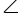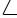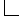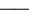To use this function, choose Calc > Calculator.

Changes degrees to radians. Trigonometric functions in the Minitab calculator require angle measures in radians. Radians and degrees are units of measure used to denote the size of an angle. The angle measure in radians equals the length of an arc when the angle vertex is at the center of a unit circle.

The measure of angle θ can also be represented in degrees. By definition, a circle has 360 degrees, so θ equals 90 degrees (90°). The following table shows angle measures represented in degrees and radians.45 π/460 π/390 π/2180 π
###### Tip

Use the Degrees function to change radians to degrees.